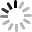# SVG 研究之路 (19) - transform 基礎篇

## translate(tx,[ty])

``````<rect fill="#c00" width="60" height="60" x="0" y="0" />
<rect fill="#09c" width="60" height="60" x="0" y="0" transform="translate(100,50)" />
``````## scale(sx,[sy])

scale 可以讓圖形放大或是縮小，若只有設定一個值，則會等比例放大縮小，若設定兩個值，則分別為水平放大或垂直放大，比較需要注意的是，scale 的放大縮小除了寬度和高度，連同坐標也會一併放大縮小，所以一開始使用的時候，會被位置跑掉給嚇到 ( 原因是因為矩陣 Matrix 運算的緣故，會在下一篇解釋 )。

``````<rect fill="#c00" width="60" height="60" x="0" y="0" />
<rect fill="#09c" width="60" height="60" x="70" y="0" transform="scale(1.2)" />
<rect fill="#0c0" width="60" height="60" x="0" y="70" transform="scale(2.5,1.3)" />
````````````<rect fill="none" width="60" height="60" x="20" y="20" stroke="#000" stroke-width="2" />
<rect fill="#c00" width="60" height="60" x="20" y="20" transform="scale(2)" />
````````````<rect fill="none" width="60" height="60" x="70" y="50" stroke="#000" stroke-width="2" />
<rect fill="#c00" width="60" height="60" x="70" y="50" transform="scale(-1,1)" />
````````````<rect fill="none" width="60" height="60" x="70" y="50" stroke="#000" stroke-width="2" />
<rect fill="#c00" width="60" height="60" x="70" y="50" transform=" translate(135) scale(-1,1)" />
````````````<rect fill="none" width="60" height="60" x="70" y="50" stroke="#000" stroke-width="2" />
<rect fill="#c00" width="60" height="60" x="70" y="50" transform="scale(-1,1) translate(-135)" />
``````## rotate(angle,[cx,cy])

rotate 可以控制圖形的旋轉角度，順時針為正，逆時針為負，旋轉圓心的預設值為 SVG 整張畫布的左上角(0,0)，當然我們也可以自訂圓心的座標值。

``````<rect fill="none" width="60" height="60" x="50" y="50" stroke="#000" stroke-width="2" />
<rect fill="#c00" width="60" height="60" x="50" y="50" transform="rotate(-20)" />
````````````<rect fill="none" width="60" height="60" x="50" y="50" stroke="#000" stroke-width="2" />
<rect fill="#c00" width="60" height="60" x="50" y="50" transform="rotate(-20,50,50)" />
``````## skewX(angle)、skewY(angle)

``````<rect fill="none" width="60" height="50" x="10" y="50" stroke="#000" stroke-width="2" />
<rect fill="#c00" width="60" height="50" x="10" y="50" transform="skewX(50)" />
````````````<rect fill="none" width="60" height="50" x="10" y="50" stroke="#000" stroke-width="2" />
<rect fill="#c00" width="60" height="50" x="10" y="50" transform="skewX(30) skewY(30)" />
``````